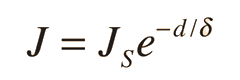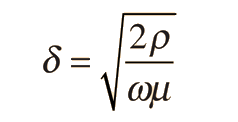# Skin Effect in AC Conduction

For Direct Current through a wire, the resistance of the wire can be calculated from its length, diameter and resistivity since it may be assumed that the electric current is essentially uniform over the cross-section of the wire. However, for Alternating Current, the interaction of electric and magnetic fields in the conductor distribute the current preferentially to the outside of the wire. This skin effect increases with frequency so that for high Radio Frequencies (RF) a thin outside layer of the conductor carries essentially all the current.

The AC current density J drops exponentially from the outside radius in a way that can be quantified in terms of a parameter called the "skin depth" δ:where JS is the current density at the surface and d is the depth beneath the surface. The skin depth δ can be calculated from:whereρ = electrical resistivity ω = angular frequency = 2π fμ = magnetic permeability
 Frequency Skin depthδ in μm Skin depthδ in mm 60 Hz 8470 8.47 10 kHz 660 0.66 100 kHz 210 0.21 1 MHz 66 0.066 10MHz 21 0.021 100 MHz 6.6 0.0066

The skin effect becomes a major consideration in RF circuits. From the exponential nature of the drop in current density, you can show that 63% of the current will flow within one skin depth δ of the surface and 98% will flow within 4δ of the surface. For frequencies above 100MHz it is wasteful of conductive metals to use solid conductors, so multiconductor straps or tubing can be used to utilize the larger surface areas.

References:

 Conductors and insulators
Index

 HyperPhysics*****Electricity and magnetism R Nave
Go Back

# Blank

Index

 HyperPhysics*****Electricity and magnetism R Nave
Go Back